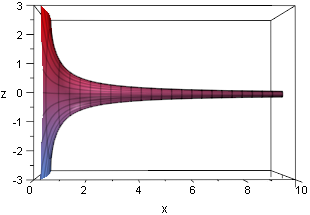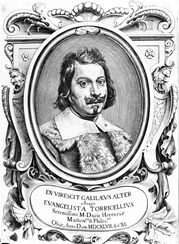# The Horn of Gabriel

Posted by Graham Wheeler on Monday, January 18, 2010

And the seventh angel sounded [his horn]; and there were great voices in heaven, saying, The kingdoms of this world are become the kingdoms of our Lord, and of his Christ; and he shall reign for ever and ever.” (Revelations 11.15, King James Edition)

In Christian and Islamic folklore, it is the angel Gabriel who is considered to be the seventh angel, who announced the coming of judgement day. An angel with such a huge responsibility clearly needs an instrument worthy of the task, and indeed there is one: Gabriel’s horn, also known as Torricelli’s trumpet, after its discoverer, Evangelista Torricelli, a student of Galileo. Gabriel’s horn has infinite surface area but finite volume, and is described the rotating the curve $$y=\frac{1}{x}$$ for $$x \geq 1$$ around the $$x$$ axis.It is easy to see that this shape, which extends to infinity along the positive $$x$$ axis but gets thinner and thinner, must have infinite surface area. It is less obvious that it has finite volume, although this can be shown with elementary calculus (if you’re unfamiliar with the calculus needed for surface area of revolution and solid volume of revolution, just bear with me).

The volume is given by:

$$V = \pi \int_{1}^{a} \frac{1}{x^2} dx = \pi (1 - \frac{1}{a})$$

While the surface area is given by:

$$A = 2 \pi \int_{1}^{a} \frac{\sqrt{1 + \frac{1}{x^4}}}{x} dx > 2 \pi \int_{1}^{a} \frac{\sqrt{1}}{x} dx = 2 \pi ln(a)$$

Clearly in the limit $$a \rightarrow \infty$$, we have:

$$V = \pi$$

$$A = \infty$$

At the time that Torricelli discovered this (1641), calculus had not yet been invented, and he obtained the results by a technique developed by his friend Cavalieri, called the summation of plane slices - essentially a technique similar to the calculus of limits using successively smaller slices. This itself required a leap of faith at the time and only became solidified later, as the idea of limits took hold, and the concept of infinitely many infinitely thin slices was given a rigorous mathematical foundation.

Torricelli’s trumpet caused some consternation and disbelief - in 1672, for example, Thomas Hobbes, the English philosopher, claimed that to believe Torricelli would be madness. It appears to result in a paradox, the painter’s paradox: if the volume is finite, the horn can be filled with a finite amount of paint, and yet to cover its interior surface would require an infinite amount of paint! Before reading on, can you resolve this paradox?

The paradox comes from confusing our mental model of real paint with “mathematical” paint. Real paint has a finite thickness - say the thickness of a paint molecule. At some point the trumpet becomes thinner than this, and so with real paint we could neither fill the trumpet nor cover its surface. The only paint that could do this would be “mathematical” paint that has infinitely small thickness. A finite amount of infinitely thin paint could cover an infinite surface.While he made a number of contributions to mathematics, Torricelli is more well known for his contributions to physics. He was the first scientist to create a sustained vacuum and suggested the experiments that led to the invention of the barometer; later he went on to use the much more effective mercury in place of water. In his honour the vacuum in a barometer is known as a Torricelli vacuum, and the Torr is a measure of vacuum. He gave the first scientific explanation for wind, which he recognized as being caused by variations in air density caused by temperature differences. He was a skilled lens maker and his telescopes and microscopes provided much of his income. Sadly, he contracted typhoid and died at the age of 39  in 1647;  not all his writing and research was preserved, and had he lived longer he may well have been credited with being the inventor of integral calculus; he was well on the way to understanding its principles.

We live submerged at the bottom of an ocean of air.” - Evangelista Torricelli, 1644.